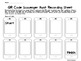# QR Code Scavenger Hunts: Grades 3 and 4 Math {Set 2}Subject
Resource Type
File Type

PDF

(5 MB|46 pages)
Standards
Also included in:
1. QR Code Scavenger Hunt BUNDLE: This is a discounted bundle (over 15% off) of the following two QR code scavenger hunt products.QR Code Math Scavenger Hunts for Grades 3 & 4 {Set 1} QR Code Math Scavenger Hunts for Grades 3 & 4 {Set 2} QR code scavenger hunts are a regular part of my own math
\$11.50
\$9.70
Save \$1.80
• Product Description
• Standards
QR Code Scavenger Hunts: Grades 3 and 4 Math (Set 2):
This file contains EIGHT separate QR code scavenger hunts that cover several crucial Common Core foundational skills in NBT and OA. These are perfect for beginning/end of year review or test prep to make sure your students have mastered the most critical Common Core math concepts. I use these simultaneously throughout the year with my other QR code scavenger hunt bundle, which covers all domains.

Each set is a different color, so you can leave several different hunts around the room without students getting confused. Students find the phone that says “Start” and scan the QR code to reveal the first question. Each QR code will lead them to the next phone until they complete the scavenger hunt. No work is required of the teacher except printing, laminating, and hiding the phones. A student response sheet (with directions) and answer keys are included.

Be sure that you have a mobile device that can scan QR codes before purchasing (iPad, iPod, iPod Touch, Smart Phone, Tablet). Haven’t taken the QR plunge yet and want to try a set for free? Click HERE

Please download the preview to see exactly what’s included in this set. Here are the following skills covered and the Common Core alignment:
-Multi-digit Addition With Regrouping 3.NBT.2, 4.NBT.4
-Multi-digit Subtraction With Regrouping 3.NBT.2, 4.NBT.4
-Multiplication (up to 4 digits x 1-digit and 2 x 2 digits) 4.NBT.5
-Division With Remainders (2-4 digit dividends and 1-digit divisors) 4.NBT.6
-Find the Missing Number in a Fact Family 3.OA.4, 3.OA.7, 4.OA.1
-Expanded to Standard Form (find the standard form of a number when given the expanded form- through the millions) 4.NBT.2
-Word to Standard Form (find the standard form of a number when given the word form- through the millions) 4.NBT.2
-Place Value: Identifying Places (name the digit in a specified place- through the millions) 4.NBT.2

*****You can now purchase this product and my other QR code scavenger hunt set as a DISCOUNTED BUNDLE.*****
Interpret a multiplication equation as a comparison, e.g., interpret 35 = 5 × 7 as a statement that 35 is 5 times as many as 7 and 7 times as many as 5. Represent verbal statements of multiplicative comparisons as multiplication equations.
Fluently multiply and divide within 100, using strategies such as the relationship between multiplication and division (e.g., knowing that 8 × 5 = 40, one knows 40 ÷ 5 = 8) or properties of operations. By the end of Grade 3, know from memory all products of two one-digit numbers.
Determine the unknown whole number in a multiplication or division equation relating three whole numbers. For example, determine the unknown number that makes the equation true in each of the equations 8 × ? = 48, 5 = __ ÷ 3, 6 × 6 = ?.
Find whole-number quotients and remainders with up to four-digit dividends and one-digit divisors, using strategies based on place value, the properties of operations, and/or the relationship between multiplication and division. Illustrate and explain the calculation by using equations, rectangular arrays, and/or area models.
Multiply a whole number of up to four digits by a one-digit whole number, and multiply two two-digit numbers, using strategies based on place value and the properties of operations. Illustrate and explain the calculation by using equations, rectangular arrays, and/or area models.
Total Pages
46 pages
Included
Teaching Duration
N/A
Report this Resource to TpT
Reported resources will be reviewed by our team. Report this resource to let us know if this resource violates TpT’s content guidelines.